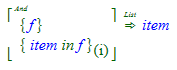# The MatchInIndexed Object

The MatchInIndexed object works the same way as the MatchIn object except that the binding includes both the term and the index of the term in the parent expression.

For the MatchIn object, this match expression:produces three binding lists. In the first list, "p" is bound to "a", in the second "p" is bound to "b" and in the third, "p" is bound to "c".

We can construct a similar expression using a MatchInIndexed object instead:In this case, we are assuming that we call our function with the same list (containing "a", "b" and "c") that we used in the previous example. We still get three binding lists, but because we are using the MatchInIndexed object, "p" is bound to { a, 0 }, { b, 1 }, { c, 2 }. In each binding the outer object is a Set object, the first item in the set is a parameter from the right-hand side of the MatchInIndexed object and the second item in the set is the index of that term in the parent expression. Parameter indexing is zero based, so the binding from the first parameter will always be { expr, 0 }.

Patterns like { p, i } can be used on the left-hand side of an indexed match in expression. For example, this clause returns parameters 1, 2, and 3 from the object bound to "f":The only difference is that now there are two bindings generated for each parameter. The first binding list would have "p" bound to "a" and "i" bound to 0, assuming that "f" is the list from our first example above.

Note that this clause will produce a result even if the expression bound to "f" has fewer than four parameters. The returned list will just have however many parameters are in the expression, excluding the first because of the i >= 1 term.